## Plotting System

The core plotting and graphics engine in R is encapsulated in the following packages:

• graphics: contains plotting functions for the “base” graphing systems, including plot, hist, boxplot and many others.

• grDevices: contains all the code implementing the various graphics devices, including X11, PDF, PostScript, PNG, etc.

The lattice plotting system is implemented using the following packages:

• lattice: contains code for producing Trellis graphics, which are independent of the вЂњbaseвЂќ graphics system; includes functions like xyplot, bwplot, levelplot

• grid: implements a different graphing system independent of the вЂњbaseвЂќ system; the lattice package builds on top of grid; we seldom call functions from the grid package directly

## The Process of Making a Plot

When making a plot one must first make a few considerations (not necessarily in this order):

• Where will the plot be made? On the screen? In a file?
• How will the plot be used?
• Is the plot for viewing temporarily on the screen?
• Will it be presented in a web browser?
• Will it eventually end up in a paper that might be printed?
• Are you using it in a presentation?

• Is there a large amount of data going into the plot? Or is it just a few points?

• Do you need to be able to dynamically resize the graphic?

## The Process of Making a Plot

• What graphics system will you use: base, lattice, or ggplot2? These generally cannot be mixed.

• Base graphics are usually constructed piecemeal, with each aspect of the plot handled separately through a series of function calls; this is often conceptually simpler and allows plotting to mirror the thought process

• Lattice graphics are usually created in a single function call, so all of the graphics parameters have to specified at once; specifying everything at once allows R to automatically calculate the necessary spacings and font sizes.

• ggplot2 combines concepts from both base and lattice graphics but uses an independent implementation

We focus on using the base plotting system to create graphics on the screen device.

## Base Graphics

Base graphics are used most commonly and are a very powerful system for creating 2-D graphics.

• There are two phases to creating a base plot
• Initializing a new plot
• Annotating (adding to) an existing plot

• Calling plot(x, y) or hist(x) will launch a graphics device (if one is not already open) and draw a new plot on the device

• If the arguments to plot are not of some special class, then the default method for plot is called; this function has many arguments, letting you set the title, x axis label, y axis label, etc.

• The base graphics system has many parameters that can set and tweaked; these parameters are documented in ?par; it wouldnвЂ™t hurt to try to memorize this help page!

## Simple Base Graphics: Histogram

library(datasets)
hist(airquality\$Ozone)  ## Draw a new plot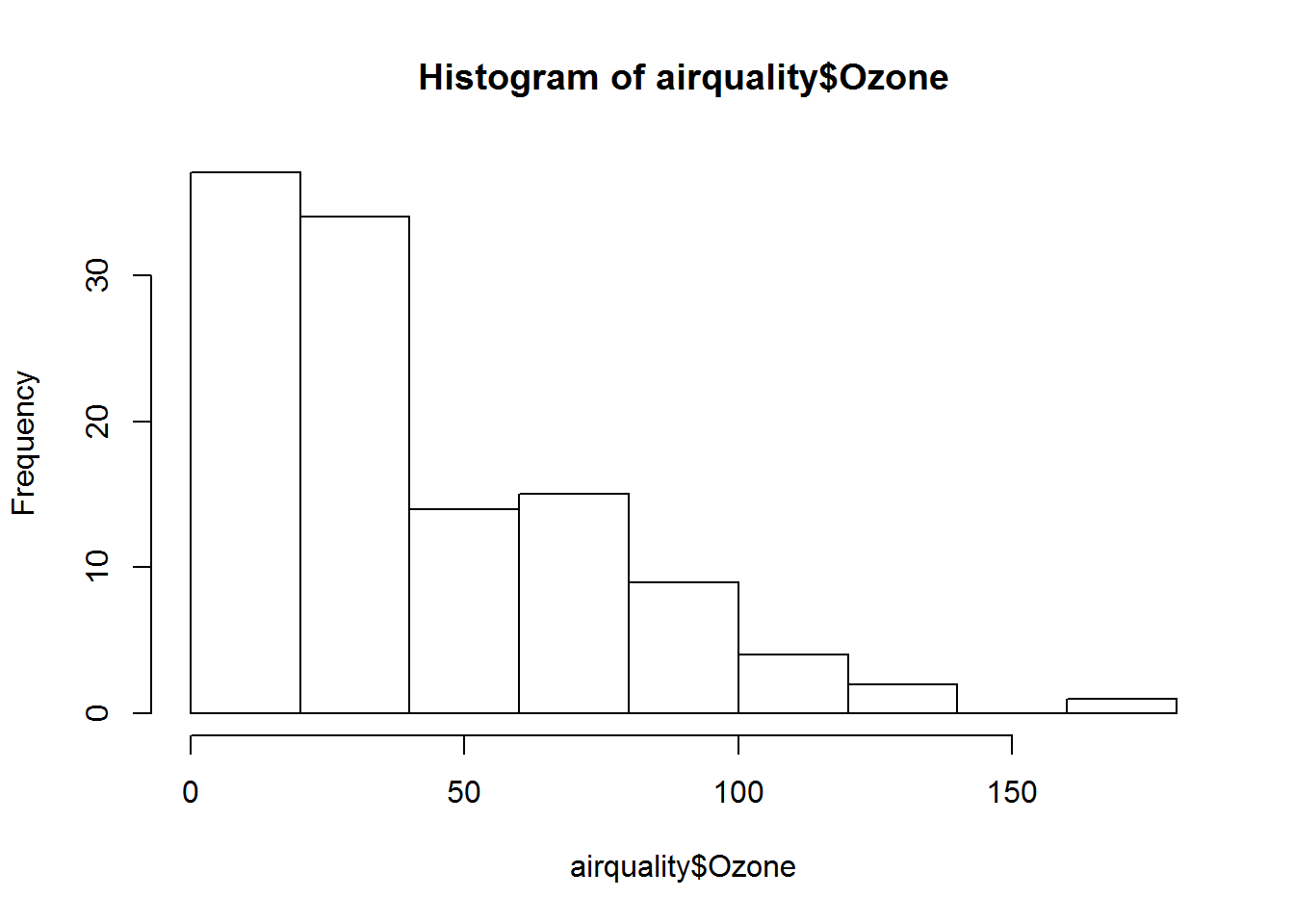## Simple Base Graphics: Scatterplot

library(datasets)
with(airquality, plot(Wind, Ozone))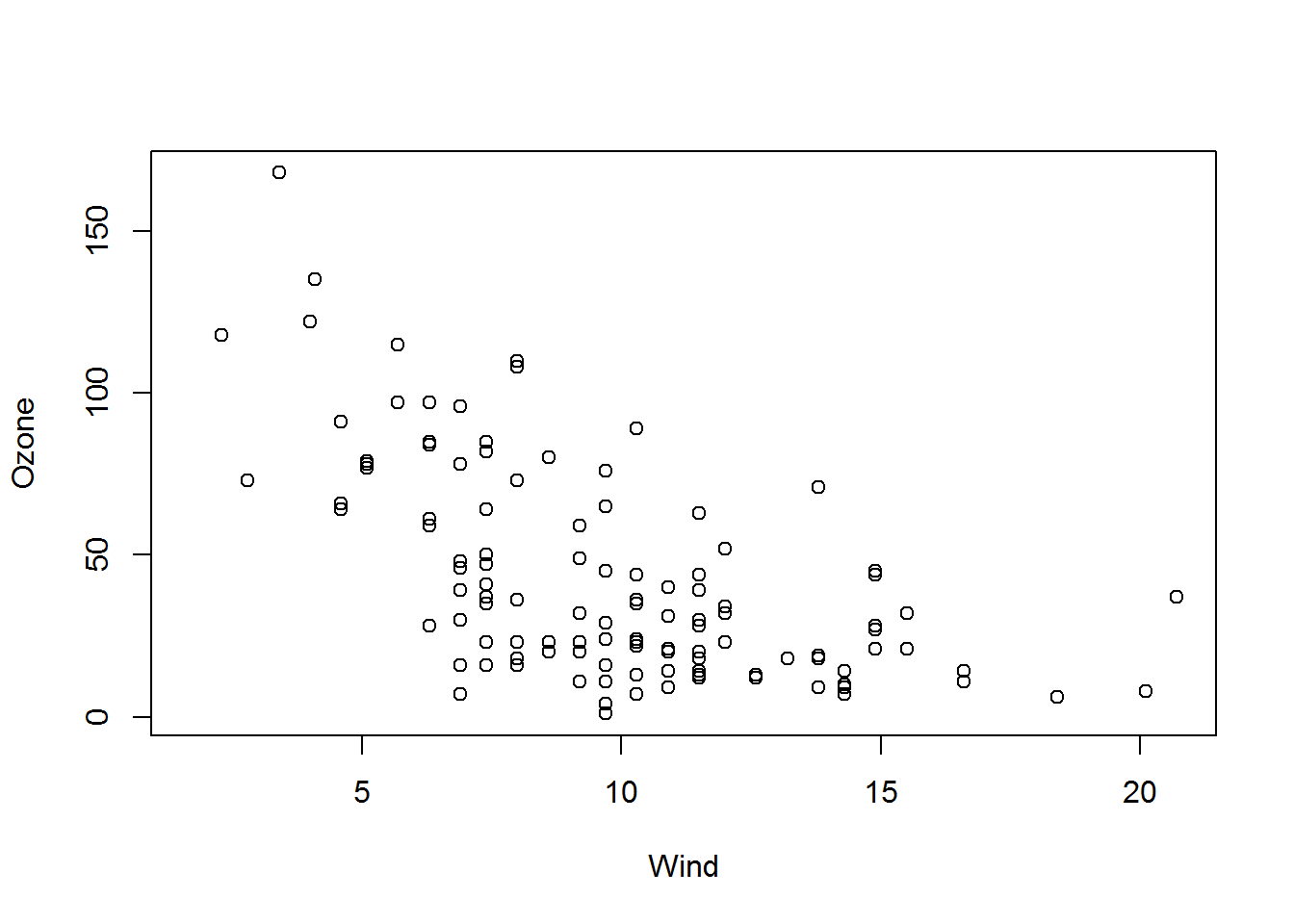## Simple Base Graphics: Boxplot

library(datasets)
airquality <- transform(airquality, Month = factor(Month))
boxplot(Ozone ~ Month, airquality, xlab = "Month", ylab = "Ozone (ppb)")

## Some Important Base Graphics Parameters

Many base plotting functions share a set of parameters. Here are a few key ones:

• pch: the plotting symbol (default is open circle)

• lty: the line type (default is solid line), can be dashed, dotted, etc.

• lwd: the line width, specified as an integer multiple

• col: the plotting color, specified as a number, string, or hex code; the colors() function gives you a vector of colors by name

• xlab: character string for the x-axis label

• ylab: character string for the y-axis label

## Some Important Base Graphics Parameters

The par() function is used to specify global graphics parameters that affect all plots in an R session. These parameters can be overridden when specified as arguments to specific plotting functions.

• las: the orientation of the axis labels on the plot
• bg: the background color
• mar: the margin size
• oma: the outer margin size (default is 0 for all sides)
• mfrow: number of plots per row, column (plots are filled row-wise)
• mfcol: number of plots per row, column (plots are filled column-wise)

## Some Important Base Graphics Parameters

Default values for global graphics parameters

par("lty")
##  "solid"
par("col")
##  "black"
par("pch")
##  1

## Some Important Base Graphics Parameters

Default values for global graphics parameters

par("bg")
##  "white"
par("mar")
##  5.1 4.1 4.1 2.1
par("mfrow")
##  1 1

## Base Plotting Functions

• plot: make a scatterplot, or other type of plot depending on the class of the object being plotted

• lines: add lines to a plot, given a vector x values and a corresponding vector of y values (or a 2-column matrix); this function just connects the dots

• points: add points to a plot
• text: add text labels to a plot using specified x, y coordinates
• title: add annotations to x, y axis labels, title, subtitle, outer margin
• mtext: add arbitrary text to the margins (inner or outer) of the plot
• axis: adding axis ticks/labels

## Base Plot with Annotation

library(datasets)
with(airquality, plot(Wind, Ozone))
title(main = "Ozone and Wind in New York City")  ## Add a title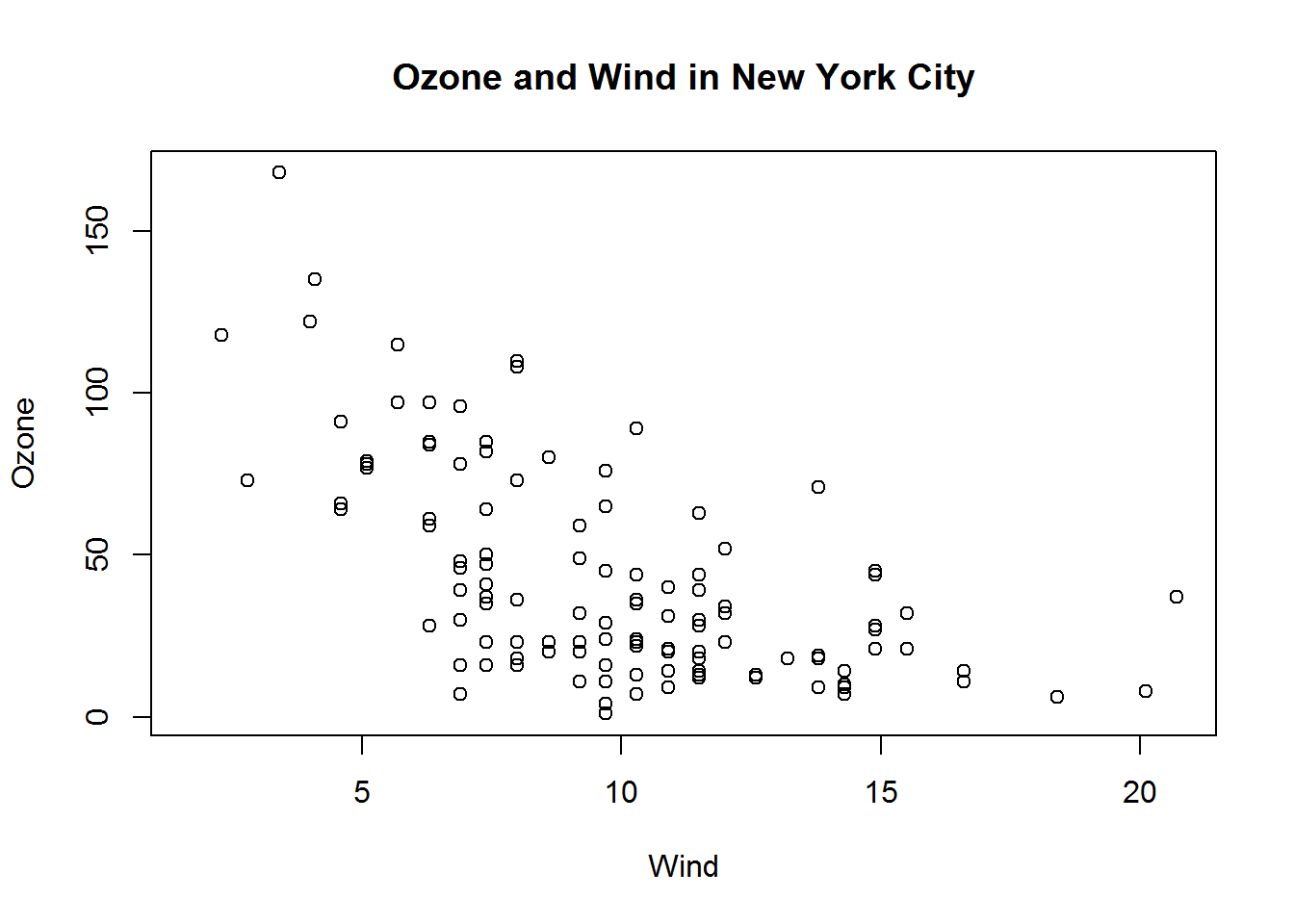## Base Plot with Annotation

with(airquality, plot(Wind, Ozone, main = "Ozone and Wind in New York City"))
with(subset(airquality, Month == 5), points(Wind, Ozone, col = "blue"))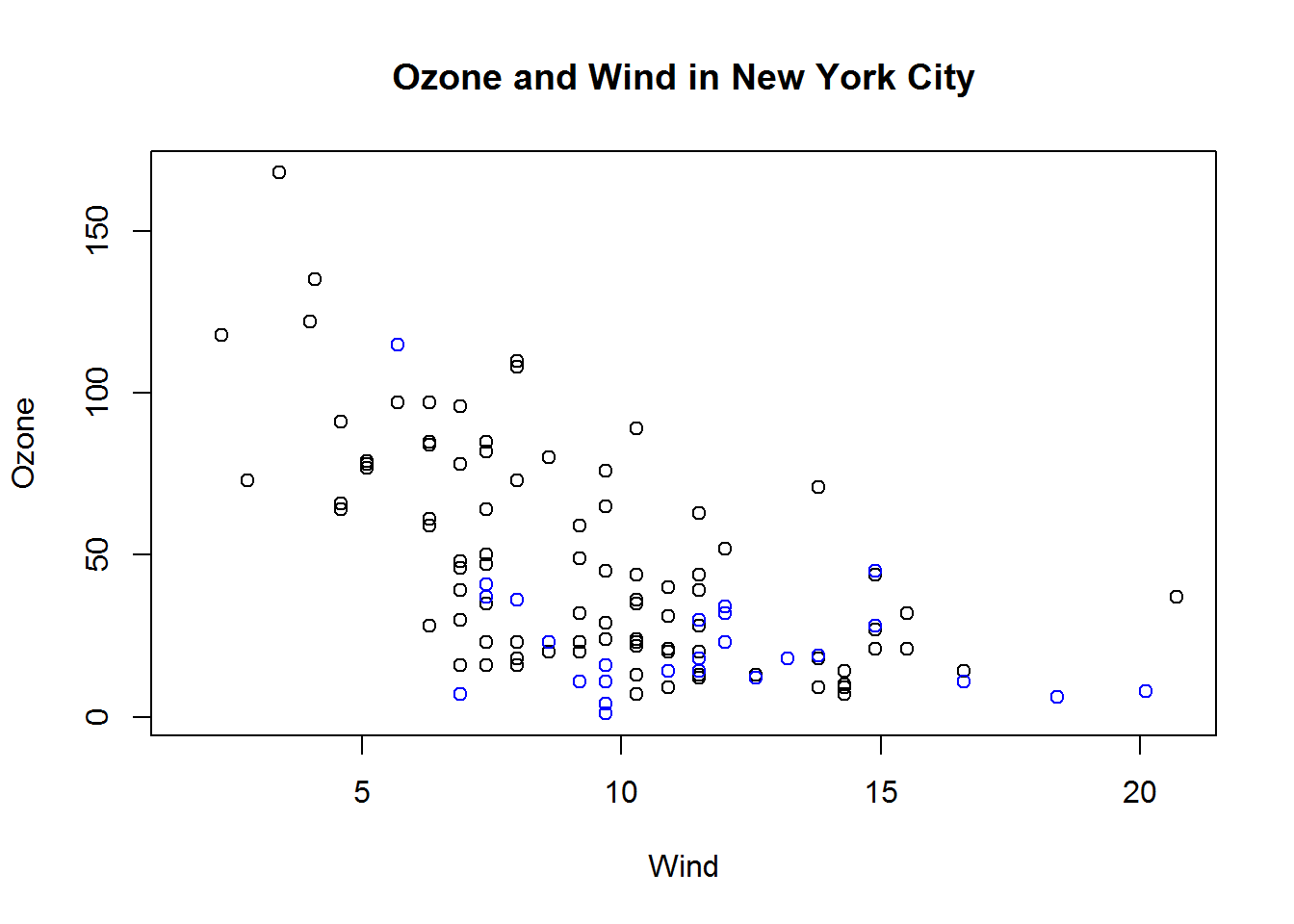## Base Plot with Annotation

with(airquality, plot(Wind, Ozone, main = "Ozone and Wind in New York City", type = "n"))
with(subset(airquality, Month == 5), points(Wind, Ozone, col = "blue"))
with(subset(airquality, Month != 5), points(Wind, Ozone, col = "red"))
legend("topright", pch = 1, col = c("blue", "red"), legend = c("May", "Other Months"))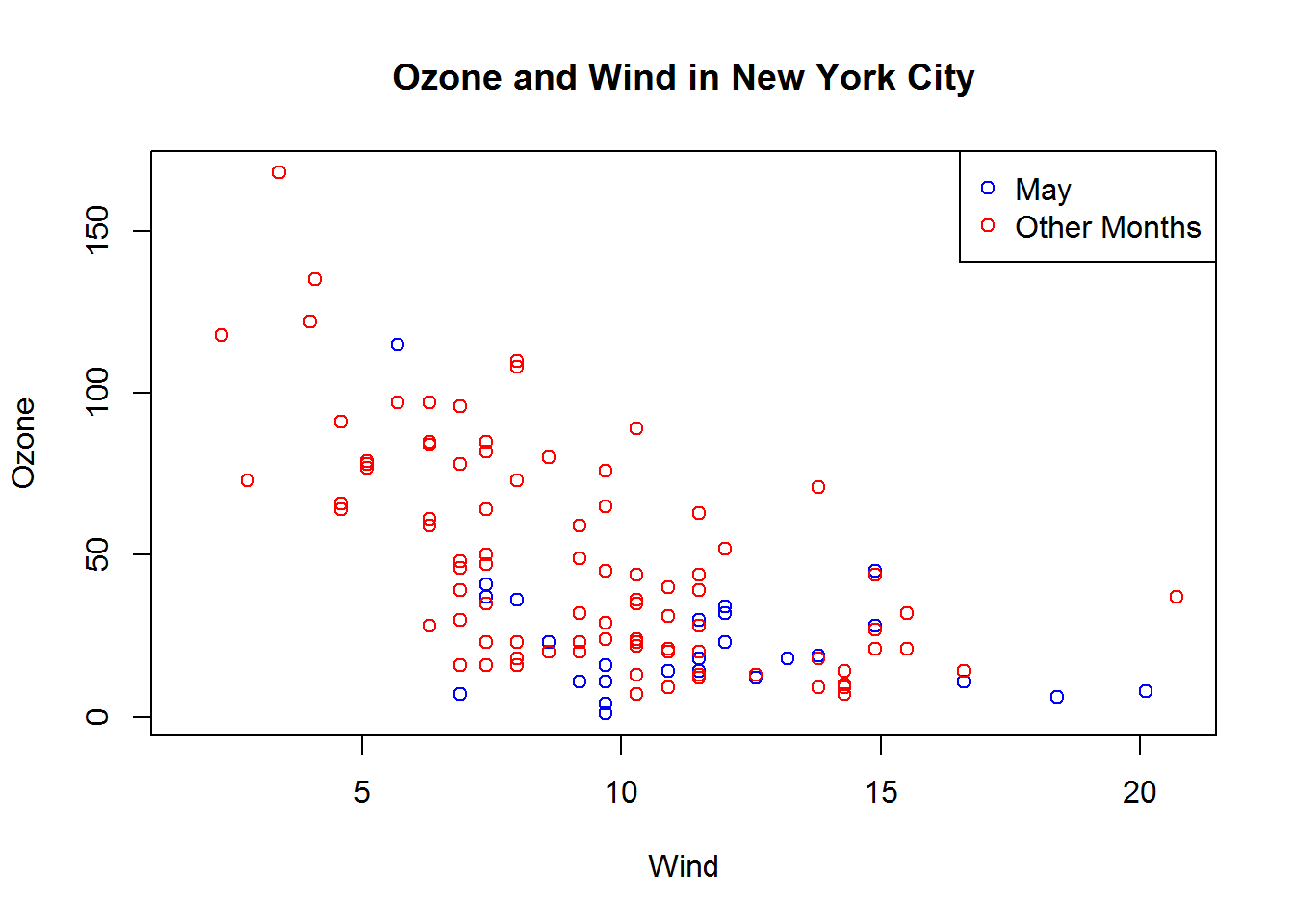## Base Plot with Regression Line

with(airquality, plot(Wind, Ozone, main = "Ozone and Wind in New York City", pch = 20))
model <- lm(Ozone ~ Wind, airquality)
abline(model, lwd = 2)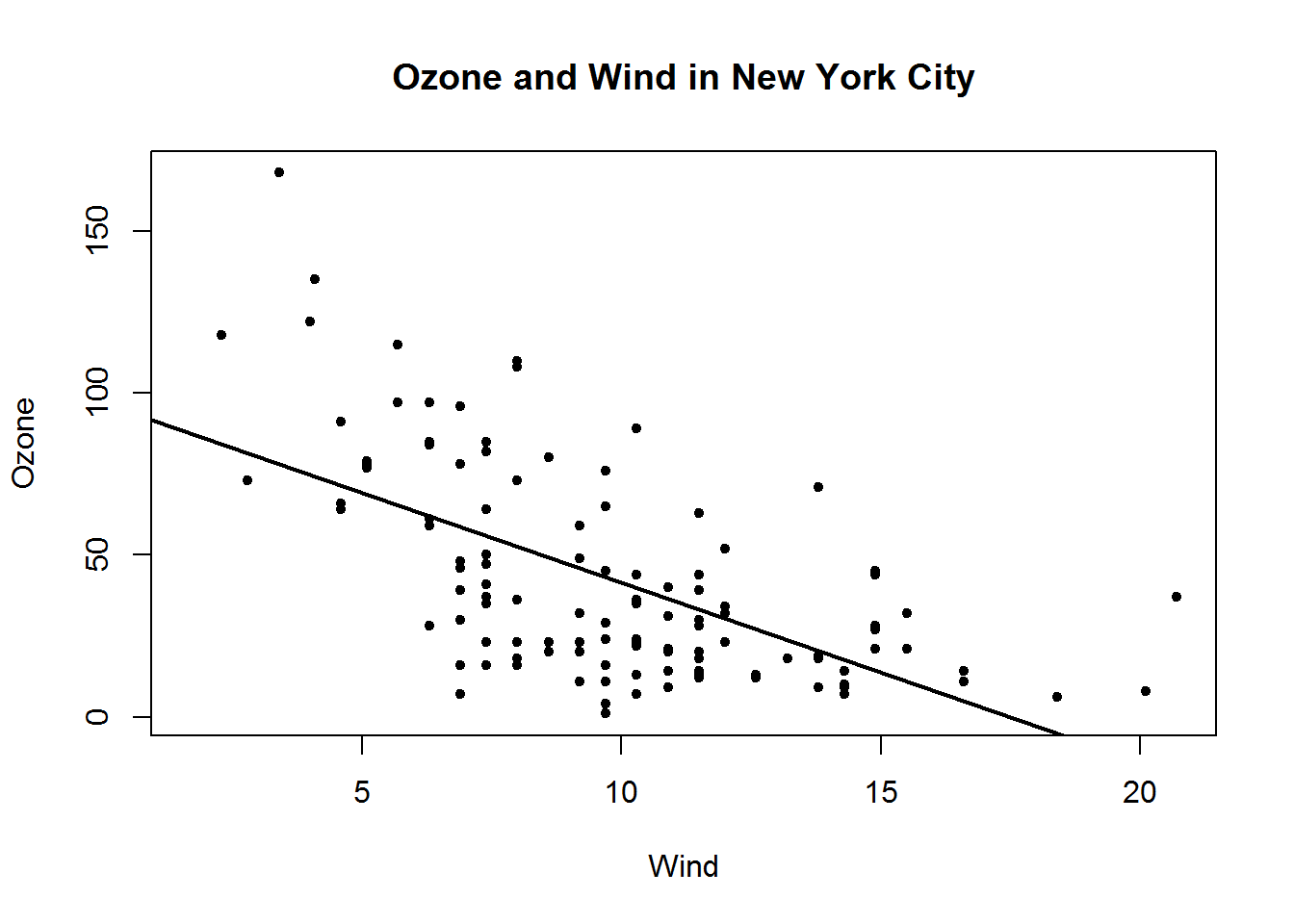## Multiple Base Plots

par(mfrow = c(1, 2))
with(airquality, {
plot(Wind, Ozone, main = "Ozone and Wind")
plot(Solar.R, Ozone, main = "Ozone and Solar Radiation")
})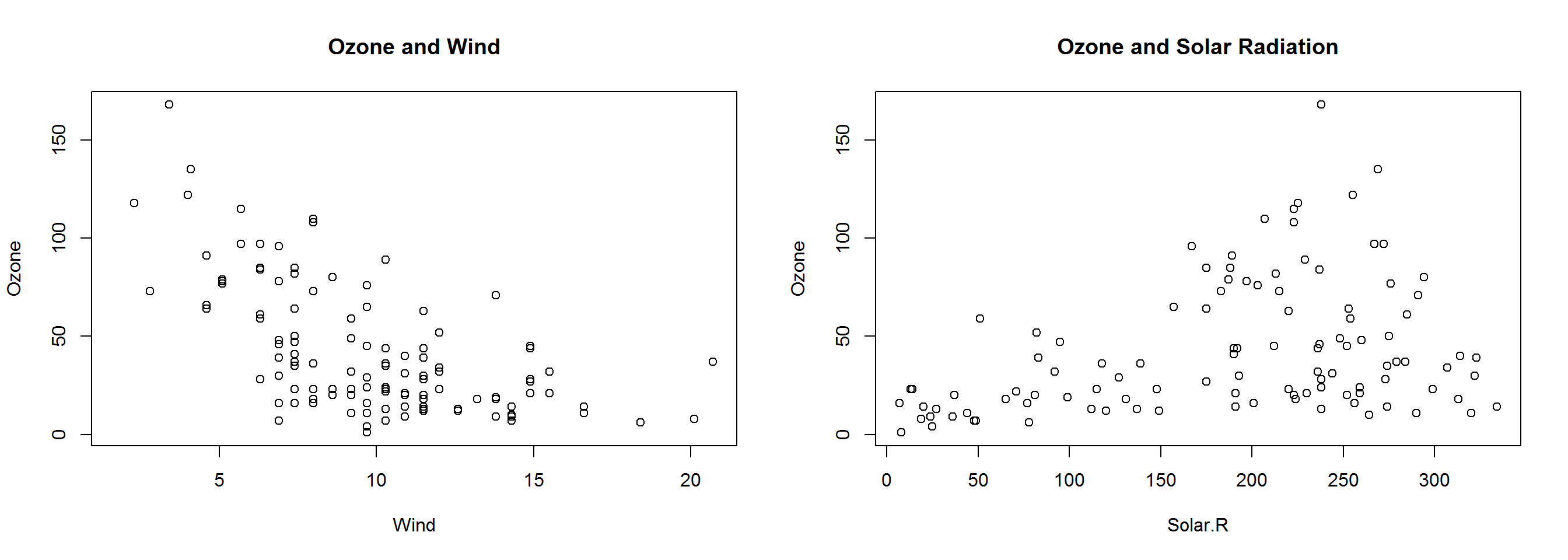## Multiple Base Plots

par(mfrow = c(1, 3), mar = c(4, 4, 2, 1), oma = c(0, 0, 2, 0))
with(airquality, {
plot(Wind, Ozone, main = "Ozone and Wind")
plot(Solar.R, Ozone, main = "Ozone and Solar Radiation")
plot(Temp, Ozone, main = "Ozone and Temperature")
mtext("Ozone and Weather in New York City", outer = TRUE)
})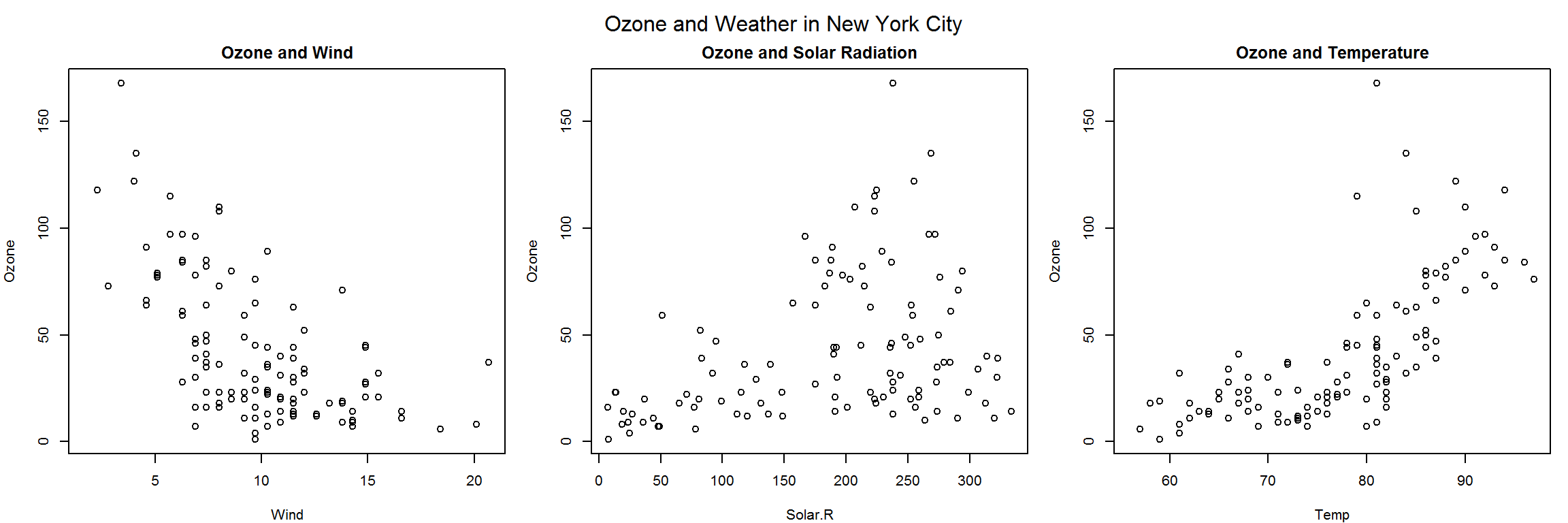## Summary

• Plots in the base plotting system are created by calling successive R functions to “build up” a plot

• Plotting occurs in two stages:
• Creation of a plot
• Annotation of a plot (adding lines, points, text, legends)

• The base plotting system is very flexible and offers a high degree of control over plotting